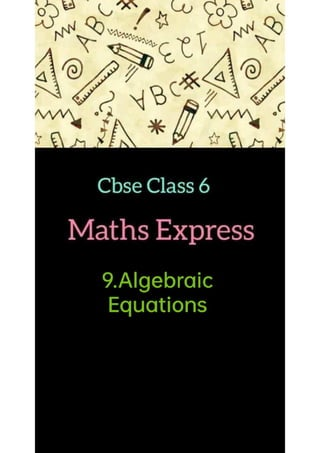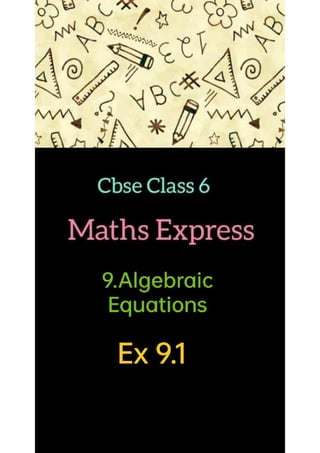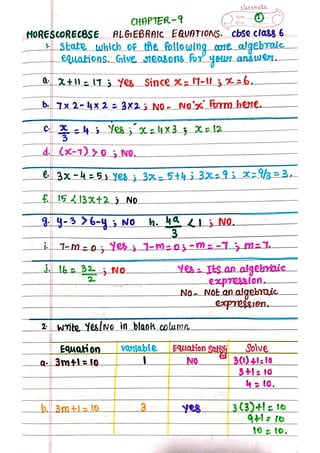Seu SlideShare está sendo baixado. ×

# akgebraic equations .pdf

Anúncio
Anúncio
Anúncio
Anúncio
Anúncio
Anúncio
Anúncio
Anúncio
Anúncio
Anúncio
AnúncioAnúncio
Anúncio
Carregando em…3
×

1 de 10 Anúncio

# akgebraic equations .pdf

Class 6 Algebraic Equations is a course that focuses on teaching students the basics of solving equations using algebraic methods. Students will learn how to use variables and mathematical operations to solve equations, as well as how to use the order of operations to simplify expressions.

Class 6 Algebraic Equations is a course that focuses on teaching students the basics of solving equations using algebraic methods. Students will learn how to use variables and mathematical operations to solve equations, as well as how to use the order of operations to simplify expressions.

Anúncio
Anúncio